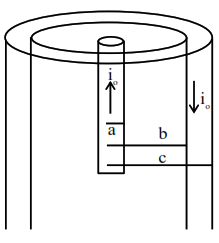# A coaxial cable consists

Question:

A coaxial cable consists of an inner wire of radius 'a' surrounded by an outer shell of inner and outer radii ' $b$ ' and 'c' respectively. The inner wire carries an electric current $\mathrm{i}_{0}$, which is distributed uniformly across cross-sectional area. The outer shell carries an equal current in opposite direction and distributed uniformly. What will be the ratio of the magnetic field at a distance $x$ from the axis when (i) $\mathrm{x}<\mathrm{a}$ and (ii) $\mathrm{a}<\mathrm{x}<\mathrm{b}$ ?

1. $\frac{x^{2}}{a^{2}}$

2. $\frac{a^{2}}{x^{2}}$

3. $\frac{x^{2}}{b^{2}-a^{2}}$

4. $\frac{b^{2}-a^{2}}{x^{2}}$

Correct Option: 1

Solution:when $x$\mathrm{B}_{1}(2 \pi \mathrm{x})=\mu_{\mathrm{o}}\left(\frac{\mathrm{i}_{\mathrm{o}}}{\pi \mathrm{a}^{2}}\right) \pi \mathrm{x}^{2}\mathrm{B}(2 \pi x)=\frac{\mu_{0} i_{0} x^{2}}{a^{2}}\mathrm{B}_{1}=\frac{\mu_{\mathrm{o}} \mathrm{i}_{\mathrm{o}} \mathrm{x}}{2 \pi \mathrm{a}^{2}}$...(1) when$\mathrm{a}<\mathrm{x}<\mathrm{b}\mathrm{B}_{2}(2 \pi \mathrm{x})=\mu_{0} \mathrm{i}_{\mathrm{o}}\mathrm{B}_{2}=\frac{\mu_{\mathrm{o}} \mathrm{i}_{\mathrm{o}}}{2 \pi \mathrm{x}}$...(2)$\frac{\mathrm{B}_{1}}{\mathrm{~B}_{2}}=\frac{\mu_{\mathrm{o}} \mathrm{i}_{\mathrm{o}} \frac{\mathrm{x}}{2 \pi \mathrm{a}^{2}}}{\frac{\mu_{\mathrm{o}} \mathrm{i}_{\mathrm{o}}}{2 \pi \mathrm{x}}}=\frac{\mathrm{x}^{2}}{\mathrm{a}^{2}}\$